# sencd.05 - Concrete fire resistance design

sencd.05

## Highlights

 Full integration into the module for the design of concrete structures. Graphical and tabular output. Three types of checks: Detailing provisions; Simplified calculation method; Advanced calculation method.

Check of the fire resistance of beams, columns and hollow core slabs according to detailing rules and the simplified method as defined in EN 1992-1-2.

The fire resistance EN 1992-1-2 is a SCIA Engineer module for checking both reinforced and prestressed concrete 1D members (beams and columns) according to EN 1992-1-2. The program is fully integrated with SCIA Engineer modules for structural analysis and with modules for checks of reinforced and prestressed concrete members according to EN 1992-1-1.

## Working with fire resistance EN 1992-1-2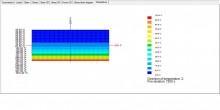SCIA Engineer system provides a graphical environment within which the fire resistance checks of reinforced and prestressed concrete cross-sections are performed in a similar way to regular concrete code checks. The members to be checked are selected graphically using the mouse. Graphical functions such as Pan, Zoom in/ out, Zoom window, user defined viewpoint, selection by the intersection line etc. make the work easy, even for complex structures. 3D structure views graphically represent the integrity check. Colours give a clear overview of any over-dimensioning and unsatisfactory parts of the structure. The output produced by the module may include:

• Automatic search for extremes: critical load case/combination, critical beam;
• Highlighting of unsatisfactory beams;
• Explanation of warning and errors that occurred during the check.

The option “single check” can be used to view the stress-strain diagram, strain and stresses over the height of the section, distribution of temperature and 3D interaction diagram.

## Input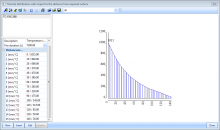Before a fire resistance check can be performed, it is necessary to define distribution temperature curves in the temperature curves library. One item of the library can contain more temperature curves and you can interpolate between them.

The temperature curve is used for the definition of the accidental thermal load with the following basic properties:

•  Side of cross-section which is exposed to fire (+Z,-Z,+Y,-Y);
•  Temperature distribution curve;
• Number of cross-section layers to be used for the integration of the temperature distribution curve required to obtain the equivalent linear temperature load and for calculation of cross-section spalling

The program offers all significant fire resistance factors and coefficients that can be further edited.

Basic fire resistance settings for fire resistance EN 1992-1-2:

• Type of calculation for the evaluation of time resistance R and critical temperature Theta_cr for detailing provisions;
• Reduction factors mu_fi for columns and detailing provisions;
• Reduction factor for design of load level for simplified method;
• Tabulated data of minimum dimensions of cross-section and minimal axial distance of reinforcement from the edge exposed to fire for basic members (beams, columns, slabs and hollow core slabs);
• Fire resistance safety factors.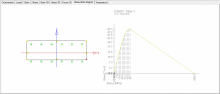In the Concrete member data, you can define the following fire resistance properties for each member:

• Type of beam used for members of type “beam” (simply supported member and continuous beam);
• Exposure conditions for type “column” (one side or more than one side);
• Type of calculation used for calculation of fire (time resistance) R and critical temperature Theta_cr for detailing provisions;
• Type of member used for prestressed members (only statically determined structures without redistribution of secondary forces caused by prestressing due to fire can be checked).

## Checks

Three types of checks have been implemented for 1D members:

• Detailing provisions (tabulated data), chapter 5;
• Simplified calculation method (zone method), annex B.2;
• Advanced calculation method.

## Detailing provisions

This type of check uses tabulated values that are for the basic types of members prescribed in the code. It checks the dimensions of the cross-section and the distance of the reinforcement from the edge of the cross-section exposed to fire. Assumptions of this approach:

• Height of the cross-section is not reduced;
• Material characteristics of the reinforcement and concrete remain unchanged;
• Temperature across the height of the cross-section can be read from the temperature curve, calculated according to the code or your input.

## Simplified calculation method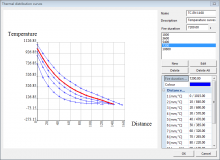This type of calculation allows for the following checks:

•        Method of limit strain;

•        Interaction diagram (resistance check).

Assumptions:

The calculation of internal forces assumes or does not take into account the following:

• Thermal expansion coefficient of concrete changes depending on the temperature (art.3.3.1(1));
• Height of the cross-section is not reduced according to the temperature;
• Material characteristics of reinforcement and concrete do not change depending on the temperature;
• Stress-strain diagrams of concrete and reinforcement do not depend on the temperature.

The following are considered in the fire resistance check:

• The height of the cross-section is reduced (zone method), annex B2 in EN 1992-1-2;
• Material characteristics of the reinforcement (table 3.2(a), 3.3) and concrete (table 3.1) change depending on the temperature;
• Stress-strain diagrams of concrete (Figure 3.1) and reinforcement (Figure 3.3) depend on the temperature.

## Advanced calculation method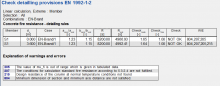This method represents a physically and geometrically nonlinear calculation that uses the following input values:

• Reduced cross-section height (zone method);Stress-strain diagrams of concrete (Figure 3.1) and reinforcement (Figure 3.3) change according to the temperature;
• Material characteristics of the reinforcement (table 3.2(a), 3.3) and concrete change (table 3.1) depend on the temperature;
• Thermal expansion coefficient of concrete calculated for the given temperature in the centre of gravity of the cross-section (art.3.3.1(1)).

## Supported cross-section and members

All types of 1D concrete members (beam, column, slabs, hollow core slabs) and concrete cross-sections can be checked using the detailing provisions and automatic calculation according to code or user-input. The calculation from temperature curve applies only to rectangular sections. The Simplified calculation method supports all non-prestressed structural members with rectangular section and statically determined prestressed structures. Advanced calculation runs only for non-prestressed members of rectangular cross-section.

Required modules:

• sen.00
Category:
Included in Edition:
Professional
Expert
Ultimate